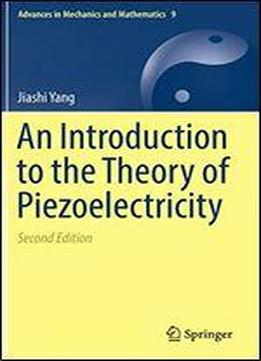# An Introduction To The Theory Of Piezoelectricity by Jiashi Yang / 2019 / English / PDF

This textbook introduces theoretical piezoelectricity. The second edition updates a classical, seminal reference on a fundamental topic that is addressed in every materials science curriculum. It presents a concise treatment of the basic theoretical aspects of continuum modeling of electroelastic interactions in solids. The general nonlinear theory for large deformations and strong fields is established and specialized to the linear theory for small deformations and weak fields, i.e., the theory of piezoelectricity. Relatively simple and useful solutions of many static and dynamic problems of piezoelectricity that are useful in device applications are given. Emphasis is on the formulation of solutions to problems rather than advanced mathematical solution techniques. This book includes many examples to assist and enhance students understanding of piezoelectricity and piezoelastics.

views: 289